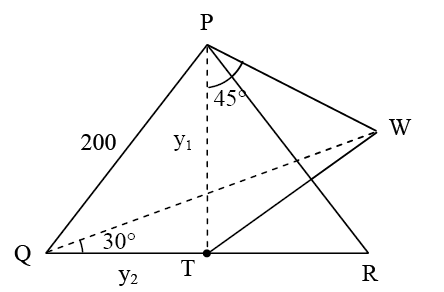PQR is a triangular park with PQ = PR = 200 m. A T.V. tower stands at the mid-point of QR...

PQR is a triangular park with PQ = PR = 200 m. A T.V. tower stands at the mid-point of QR. If the angles of elevation of the top of the tower at P, Q and R are respectively 450, 300 and 300, then the height of the tower (in m) is :

a) 100√3

b) 50√2

c) 100

d) 50

Solution:Let TW = h

ΔPTW, tan 450 = h/y1

h = y1

Similarly, h/y2 = tan 300

y2 = √3h

ΔPQT, PQ2 = QT2+PT2

40000 = 3h2+h2

4h2 = 40000

h = 100(1)(0)